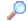Search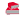Directory

Desktop Functions:

Smart Device Functions: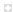Glossary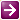Show Recent ChangesSubscribe (RSS)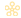Misc. Pages
HitTestValues (Structures)

Summary
TODO - a short description

#### C# Definition:

enum HitTestValues
{
HTERROR = -2,
HTTRANSPARENT = -1,
HTNOWHERE = 0,
HTCLIENT = 1,
HTCAPTION = 2,
HTGROWBOX = 4,
HTHSCROLL = 6,
HTVSCROLL = 7,
HTMINBUTTON = 8,
HTMAXBUTTON = 9,
HTLEFT = 10,
HTRIGHT = 11,
HTTOP = 12,
HTTOPLEFT = 13,
HTTOPRIGHT = 14,
HTBOTTOM = 15,
HTBOTTOMLEFT = 16,
HTBOTTOMRIGHT = 17,
HTBORDER = 18,
HTOBJECT = 19,
HTCLOSE = 20,
HTHELP = 21
}

enum HitTestValues
{
/// <summary>
/// In the border of a window that does not have a sizing border.
/// </summary>
HTBORDER = 18,

/// <summary>
/// In the lower-horizontal border of a resizable window (the user can click the mouse to resize the window vertically).
/// </summary>
HTBOTTOM = 15,

/// <summary>
/// In the lower-left corner of a border of a resizable window (the user can click the mouse to resize the window diagonally).
/// </summary>
HTBOTTOMLEFT = 16,

/// <summary>
/// In the lower-right corner of a border of a resizable window (the user can click the mouse to resize the window diagonally).
/// </summary>
HTBOTTOMRIGHT = 17,

/// <summary>
/// In a title bar.
/// </summary>
HTCAPTION = 2,

/// <summary>
/// In a client area.
/// </summary>
HTCLIENT = 1,

/// <summary>
/// In a Close button.
/// </summary>
HTCLOSE = 20,

/// <summary>
/// On the screen background or on a dividing line between windows (same as HTNOWHERE, except that the DefWindowProc function produces a system beep to indicate an error).
/// </summary>
HTERROR = -2,

/// <summary>
/// In a size box (same as HTSIZE).
/// </summary>
HTGROWBOX = 4,

/// <summary>
/// In a Help button.
/// </summary>
HTHELP = 21,

/// <summary>
/// In a horizontal scroll bar.
/// </summary>
HTHSCROLL = 6,

/// <summary>
/// In the left border of a resizable window (the user can click the mouse to resize the window horizontally).
/// </summary>
HTLEFT = 10,

/// <summary>
/// </summary>

/// <summary>
/// In a Maximize button.
/// </summary>
HTMAXBUTTON = 9,

/// <summary>
/// In a Minimize button.
/// </summary>
HTMINBUTTON = 8,

/// <summary>
/// On the screen background or on a dividing line between windows.
/// </summary>
HTNOWHERE = 0,

/// <summary>
/// Not implemented.
/// </summary>
/* HTOBJECT = 19, */

/// <summary>
/// In a Minimize button.
/// </summary>
HTREDUCE = HTMINBUTTON,

/// <summary>
/// In the right border of a resizable window (the user can click the mouse to resize the window horizontally).
/// </summary>
HTRIGHT = 11,

/// <summary>
/// In a size box (same as HTGROWBOX).
/// </summary>
HTSIZE = HTGROWBOX,

/// <summary>
/// In a window menu or in a Close button in a child window.
/// </summary>

/// <summary>
/// In the upper-horizontal border of a window.
/// </summary>
HTTOP = 12,

/// <summary>
/// In the upper-left corner of a window border.
/// </summary>
HTTOPLEFT = 13,

/// <summary>
/// In the upper-right corner of a window border.
/// </summary>
HTTOPRIGHT = 14,

/// <summary>
/// In a window currently covered by another window in the same thread (the message will be sent to underlying windows in the same thread until one of them returns a code that is not HTTRANSPARENT).
/// </summary>
HTTRANSPARENT = -1,

/// <summary>
/// In the vertical scroll bar.
/// </summary>
HTVSCROLL = 7,

/// <summary>
/// In a Maximize button.
/// </summary>
HTZOOM = HTMAXBUTTON,
}

#### VB Definition:

Enum HitTestValues
HTERROR = -2
HTTRANSPARENT = -1
HTNOWHERE = 0
HTCLIENT = 1
HTCAPTION = 2
HTGROWBOX = 4
HTHSCROLL = 6
HTVSCROLL = 7
HTMINBUTTON = 8
HTMAXBUTTON = 9
HTLEFT = 10
HTRIGHT = 11
HTTOP = 12
HTTOPLEFT = 13
HTTOPRIGHT = 14
HTBOTTOM = 15
HTBOTTOMLEFT = 16
HTBOTTOMRIGHT = 17
HTBORDER = 18
HTOBJECT = 19
HTCLOSE = 20
HTHELP = 21
End Enum

None.

#### Notes:

None.

Documentation

Do you have...

• corrections to the existing content?
• alternate definitions?
• additional languages you want to include?

Select "Edit This Page" on the right hand toolbar and edit it! Or add new pages containing any supporting types needed.Access PInvoke.net directly from VS:Terms of UseEdit This PageFind ReferencesShow Printable Version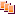Revisions Show changes